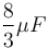Courses

# Capacitance MCQ Level - 2

## 10 Questions MCQ Test Topic wise Tests for IIT JAM Physics | Capacitance MCQ Level - 2

Description
This mock test of Capacitance MCQ Level - 2 for Physics helps you for every Physics entrance exam. This contains 10 Multiple Choice Questions for Physics Capacitance MCQ Level - 2 (mcq) to study with solutions a complete question bank. The solved questions answers in this Capacitance MCQ Level - 2 quiz give you a good mix of easy questions and tough questions. Physics students definitely take this Capacitance MCQ Level - 2 exercise for a better result in the exam. You can find other Capacitance MCQ Level - 2 extra questions, long questions & short questions for Physics on EduRev as well by searching above.
QUESTION: 1

### In the figure shown a parallel plate capacitor has a dielectric of width d/2 and dielectric constant K = 2. The other dimensions of the dielectric are same as that of the plates. The plates P1 and P2 the capacitor have area A each. The energy of the capacitor is :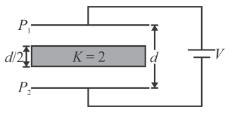Solution: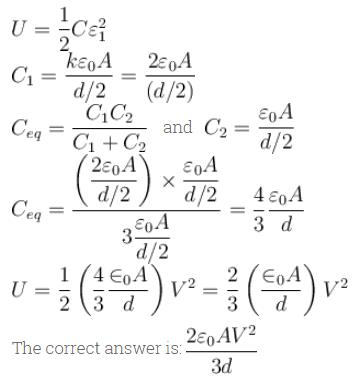QUESTION: 2

### In the circuit shown, switch S2 is closed first and is kept closed for a long time. Now S1 is closed. Just after that instant the current through S1 is :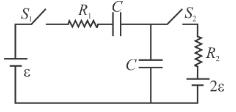Solution: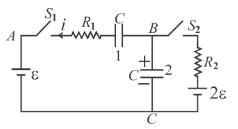just before S1 is closed the potential difference across capacitor 2 is 2ε.
just after S1 is closed the potential difference across capacitors 1 and 2 are 0 and 2ε respectively.
Applying KVL to loop ABCD immediately after S1 is closed.
ε = – iR1 + 0 + 2εThe correct answer is: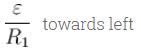QUESTION: 3

### A capacitor of capacitance 0.1µF is connected to a battery of emf 8V as shown in the figure. Under steady state condition.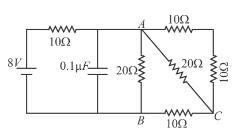Solution:

The equivalent circuit is as shown in figure (b).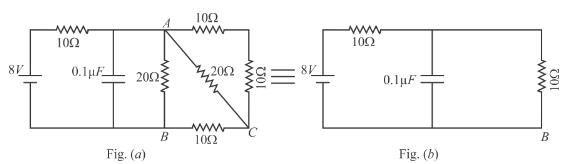In the steady state the potential difference across AB is 4 volts.
∴ Charge on capacitor in steady state is q = CV = 0.4µC
Current through resistor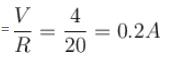The correct answer is: Charge on the capacitor is 0.4μC

QUESTION: 4

In the figure a capacitor of capacitance 2µF is connected to a cell of emf 20volt. The plates of the capacitor are drawn apart slowly to double the distance between them. The work done by the external agent on the plates is :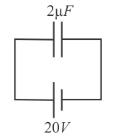Solution:

Force between plates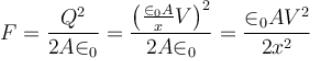where x is separation between plates
dW = Fdx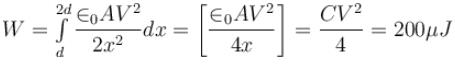QUESTION: 5

In the figure shown the plates of a parallel plate capacitor have unequal charges. Its capacitance is CP is a point outside the capacitor and close to the plate of charge Q. The distance between the plates is d select incorrect alternative :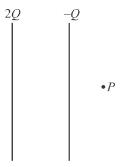Solution: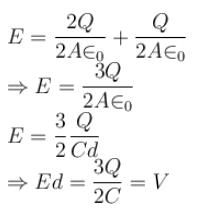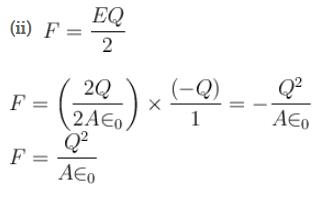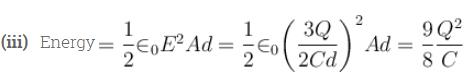The correct answer is: The force on one plate due to the other plate is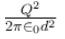QUESTION: 6

The plates S and T of an uncharged parallel plate capacitor are connected across a battery. The battery is then disconnected and the charged plates are now connected in a system as shown in the figure. The system shown is in equilibrium. All the strings and springs are insulating and massless. The magnitude of charge on one of the capacitor plate is :
(Area of plate = A)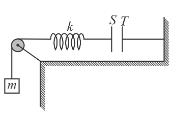Solution:

Force on metal plate S due to electrostatic attraction by plate  is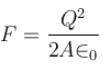Force exerted on plate S by spring is = mg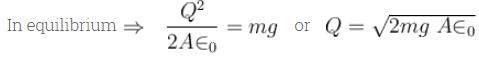The correct answer is: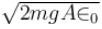QUESTION: 7

The key K (figure) is connected in turn to each of the contacts over short identical time intervals so that the change in the charge on the capacitor over each connection is small. The final charge qr on the capacitor is :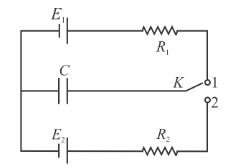Solution:

As the key is connected to 1 and 2 frequently and at equal intervals, the two emf’s Eand E2 behave as dc sources in continuous contact.
The potential due to the two cells is :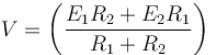Hence the charge on the capacitor is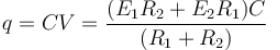The correct answer is: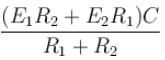QUESTION: 8

A parallel plate capacitor of capacitance C (without dielectrics) is filled by dielectric slabs as shown in the figure. Then the new capacitance of the capacitor is :Solution: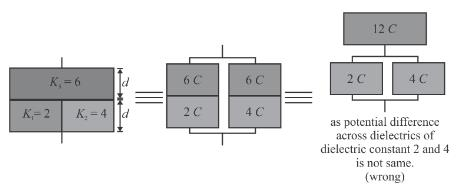The equivalent capacitance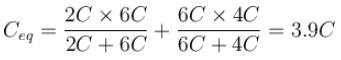QUESTION: 9

Initially switch S is connected to position 1 for a long time. The net amount of heat generated in the circuit after it is shifted to position 2 is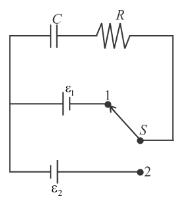Solution: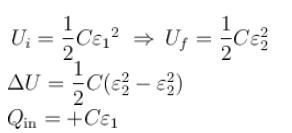work done by battery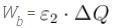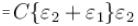Heat generated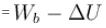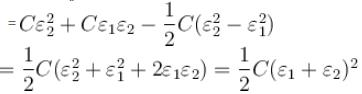The correct answer is: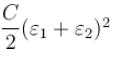QUESTION: 10

The equivalent capacitance between x and y is :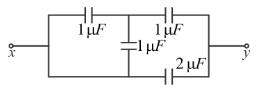Solution:

Rearrange the circuit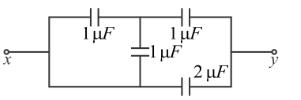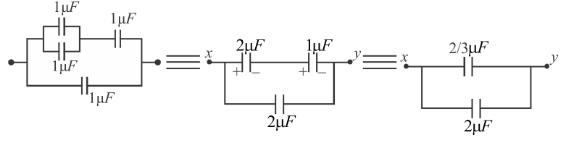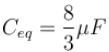The correct answer is: